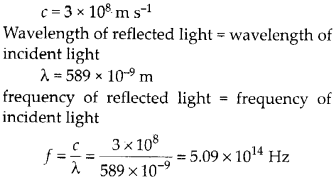Enlightened

# Question 1: NCERT Solutions for 12th Class Physics: Chapter 10-Wave Optics

• 0

Question 1: NCERT Solutions for 12th Class Physics: Chapter 10-Wave Optics

Monochromatic light of wavelength 589 nm is incident from air on a water surface. What are the wavelength, frequency and speed of
(a) reflected, and
(b) refracted light? Refractive index of water is 1.33.

Share

1. Solution:
(a) In the process of reflection wavelength, frequency and speed of incident light remain unchanged. So, speed of reflected light = speed of incident light(b) In the process of refraction wavelength and speed changes but the frequency remain the same. Speed of light in waterCheck the complete chapter with solutions.

NCERT Solutions for 12th Class Physics: Chapter 10-Wave Optics

• 0